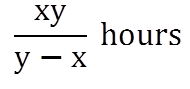# Pipes & Cistern

1) If an inlet connected to a tank fills it in X hours, part of the tank filled in one hour is = 1/X

2) If an outlet connected to a tank empties it in Y hours, part of the tank emptied in one hour is = 1/Y

3) An inlet can fill a tank in X hours and an outlet can empty the same tank in Y hours. If both the pipes are opened at the same time and Y > X, the net part of the tank filled in one hour is given by;

= (1/X – 1/Y)

Therefore, when both the pipes are open the time taken to fill the whole tank is given by;

= (XY/Y-X) Hours

If X is greater than Y, more water is flowing out of the tank than flowing into the tank. And, the net part of the tank emptied in one hour is given by;

= (1/Y – 1/X)

Therefore, when both the pipes are open the time taken to empty the full tank is given by;

= (YX/X-Y) Hours

4) An inlet can fill a tank in X hours and another inlet can fill the same tank in Y hours. If both the inlets are opened at the same time, the net part of the tank filled in one hour is given by;

= (1/X + 1/Y)

Therefore, the time taken to fill the whole tank is given by;

= (XY/Y+X) Hours

In a similar way, If an outlet can empty a tank in X hours and another outlet can empty the same tank in Y hours, the part of the tank emptied in one hour when both the pipes start working together is given by;

= (1/X + 1/Y)

1. If a pipe can fill a tank in a hrs, then the part filled in 1 hr =1/a.
2. If a pipe can empty a tank in b hrs, then the part of the full tank emptied in 1 hr = 1/b.
3. If a pipe can fill a tank in a hrs and the another pipe can empty the full tank in b hrs, then the net part filled in 1 hr, when both the pipes are opened =[1/a – 1/b] ∴ Time taken to fill the tank, when both the pipes are opened = ab/(b – a)
4. If a pipe can fill a tank in a hrs and another can fill the same tank in b hrs, then the net part filled in 1 hr, when both pipes are opened = [1/a + 1/b] ∴ Time taken to fill the tank = ab/(a + b)
5. If a pipe fills a tank in a hrs and another fills the same tank in b hrs, but a third one empties the full tank in c hrs, and all of them are opened together, the net part filled in 1 hr = [1/a+ 1/b-1/c] ∴ Time taken to fill the tank =abc/(bc + ac – ab) hrs.
6. A pipe can fill a tank in a hrs. Due to a leak in the bottom it is filled in b hrs. If the tank is full, the time taken by the leak to empty the tank = ab/(b – a) hrs.

## Basic Formulas of Pipes and Cisterns

1. If an inlet pipe can fill the tank in x hours, then the part filled in 1 hour = 1/x
2. If an outlet pipe can empty the tank in y hours, then the part of the tank emptied in 1 hour = 1/y
3. If both inlet and outlet valves are kept open, then the net part of the tank filled in 1 hour is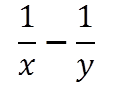## Shortcut Methods for Pipes and Cisterns

Rule 1: Two pipes can fill (or empty) a cistern in x and y hours while working alone. If both pipes are opened together, then the time taken to fill (or empty) the cistern is given by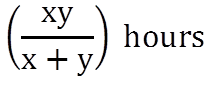Rule 2: Three pipes can fill (or empty) a cistern in x, y and z hours while working alone. If all the three pipes are opened together, the time taken to fill (or empty) the cistern is given by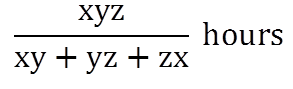Rule 3: If a pipe can fill a cistern in x hours and another can fill the same cistern in y hours, but a third one can empty the full tank in z hours, and all of them are opened together, then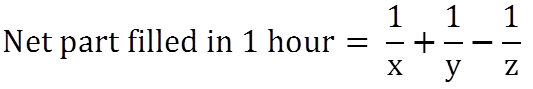Rule 4: A pipe can fill a cistern in x hours. Because of a leak in the bottom, it is filled in y hours. If it is full, the time taken by the leak to empty the cistern is